Impact Factor 1.895 | CiteScore 2.24
More on impact ›

# Frontiers in Physics## Original Research ARTICLE

Front. Phys., 13 February 2020 | https://doi.org/10.3389/fphy.2020.00010

# A Bayesian Approach to the Naming Game Model

• NICPB–National Institute of Chemical Physics and Biophysics, Tallinn, Estonia

We present a novel Bayesian approach to semiotic dynamics, which is a cognitive analog of the naming game model restricted to two conventions. The model introduced in this paper provides a general framework for studying the combined effects of cognitive and social dynamics. The one-shot learning that characterizes the agent dynamics in the basic naming game is replaced by a word-learning process in which agents learn a new word by generalizing from the evidence garnered through pairwise-interactions with other agents. The principle underlying the model is that agents—like humans—can learn from a few positive examples and that such a process is modeled in a Bayesian probabilistic framework. We show that the model presents some analogies with the basic two-convention naming game model but also some relevant differences in the dynamics, which we explain through a geometric analysis of the mean-field equations.

## 1. Introduction

A basic question in complexity theory is how the interactions between the units of the system lead to the emergence of ordered states from initially disordered configurations [1, 2]. This general question can concern different phenomena ranging from phase transitions in condensed matter systems and self-organization in living matter to the appearance of norm conventions and cultural paradigms in social systems. In order to study social interactions and cooperation, different models have been used: from those based on analogies with condensed matter systems (such as spin systems) or statistical mechanical models (e.g., using a master equation approach) to those formally equivalent to ecological competition models  or many-agents models in a game-theoretical framework . Among the various models, opinion dynamics and cultural spreading models represent an example of a valuable theoretical framework for a quantitative description of the emergence of social consensus .

Within the spectrum of phenomena associated with consensus dynamics, the emergence of human language remains a challenging question because of its multi-fold nature, characterized by biological, ecological, social, logical, and cognitive aspects . Language dynamics [11, 12] has provided a set of models describing various phenomena of language competition and language change in a quantitative way, focusing on the mutual interactions of linguistic traits (such as sounds, phonemes, grammatical rules, or the use of languages understood as fixed entities), possibly under the influence of ecological and social factors, modeling such interactions through analogy with biological competition and evolution.

However, even the basic learning process of a single word has a complex dynamics due to the associated cognitive dimension: to learn a word means to learn both a concept, understood as a pointer to a subset of objects [10, 13, 14], and a corresponding linguistic label, for example the name used for communicating the concept. The double concept↔name nature of words has been studied in semiotic dynamics models, which study the consensus dynamics of language, i.e., if and how consensus about the use of certain names to refer to a certain object-concept emerges in a group of N interacting agents.

Examples of semiotic models are those of Hurford  and Nowak et al.  (see also [17, 18]). In the basic version of the model of Nowak et al. , the language spoken by each agent i (i = 1, …, N) is defined by two personal matrices, ${U}$(i) and H(i), representing the links of a bipartite network joining Q names and R concepts: (1) the active matrix ${U}$(i) represents the concept → name links, where the element ${{U}}_{q,r}^{\left(i\right)}$ (q ∈ (1, Q), r ∈ (1, R)) gives the probability that agent i will utter the qth name to communicate the rth concept; (2) the passive matrix H(i) represents the name → concept links, in which the element ${{H}}_{q,r}^{\left(i\right)}$ represents the probability that an agent interprets the qth name as referring to the rth concept. In Hurford's and Nowak's models, the languages (i.e., the active and passive matrices) of each individual evolve over time according to a game-theoretical dynamics in which agents gain a reproductive advantage if their matrices are associated with a higher communication efficiency. These studies have achieved interesting results, showing, e.g., that the system self-organizes in an optimal way with only non-ambiguous one-to-one links between objects and sounds, when possible, and explaining why homonyms are more frequent than synonyms .

Another example of a semiotic model is the naming game (NG) model [19, 41], detailed below, where only one concept is considered (R = 1) together with its links to a set of Q > 1 different names. It is possible to reformulate the model through the lists of the name↔concept connections ${L}$i known to each agent i rather than in terms of the matrices ${U}$(i) and H(i). In the case of the NG with two names A and B, the list of the generic ith agent can be ${L}$i = ∅ if no such connection is known, ${L}$i = (A) or ${L}$i = (B), if only one name is known to refer to concept C, or ${L}$i = (A, B) if both name↔concept connections are known. At variance with Hurford's and Nowak's models, in the basic NG model, there is no population dynamics, and consensus is achieved through horizontal interactions between pairs of agents, who carry out a negotiation dynamics in which they may agree on the use of a word, possibly erasing the other word from their lists.

In the signaling game of Lenaerts et al. , the basic add/remove agreement dynamics of the NG model is replaced by a reinforcement scheme describing an underlying cognitive dynamics. Such a scheme is defined within a learning automata framework in which the single probabilities, linking the qth word and the rth object, are updated in time depending on the outcome of pair-wise communications—the system is characterized by the same complex landscape of R concepts and Q names as in Hurford's and Nowak's models. The model works with a basic horizontal dynamics, as in the NG model, but it has a general framework of language change, which can include oblique (teacher↔student) and vertical (parent↔offspring) communications. An NG-like language dynamics, with a similar cognitive reinforcement mechanism, was also studied by Lipowska & Lipowski, both in the single- and the many-object version . They also studied how the underlying topology, e.g., a random network or a regular lattice, can have a crucial role in determining the type of final state, characterized by a global consensus or by different types of local consensus fragmented into patches.

In the models mentioned, words and concepts are fixed, though their links are dynamically determined through the interactions between agents. To make further extensions of such semiotic dynamics models toward a cognitive direction is not a trivial task, both because of the complexity of the problem—for example, a two-opinion variant of the NG model that takes into account committed groups produces a remarkable phase diagram —and because, in order to describe mathematically actual cognitive effects, entirely new features need to be taken into account . A natural framework is represented by Bayesian inference, both for its general analogy with actual learning processes and especially because supported by various experiments. For example, Bayes inference underlies the agent-based model of binary decision-making introduced by , which is shown to interpolate well some real datasets on binary option choices. See Pérez et al.  for another example of Bayes-based modeling and reproduction of a real decision-making experiment.

The goal of the present paper is to construct a minimal model to study the interplay of cognitive and social dynamical dimensions. The new model (see section 2.3) is similar to the two-conventions NG but contains relevant differences that describe the cognitive dimension of word-learning. Using semiotic dynamics models as a starting point is a natural choice, and the NG is a convenient framework due to its simple yet general underlying idea, which allows applications to the emergence of different conventions. Furthermore, the NG can be coupled to various underlying processes, such as mutations, population growth, and ecological constraints, and can be easily embedded in the topology of a complex network [19, 26]. The cognitive extension of the NG is done within the experimentally validated Bayesian framework of Tenenbaum  (see also [13, 14, 2730]). In the resulting cognitive framework, an individual can learn a concept from a small number of examples, a very remarkable feature of human learning [10, 31, 32], in contrast with machine learning algorithms, which require a large number of examples to generalize successfully . In section 3, we present and discuss the features of the semiotic dynamics emerging from the numerical simulations and quantitatively compare them with those of the two-conventions NG model. It is shown that while the Bayesian NG model always reaches consensus, like the basic NG, the corresponding dynamics presents relevant differences related to the probabilistic learning process. We study in detail the stability and the other novel features of the dynamics in section 4. A summary of the work and a discussion of other possible outcomes to be expected from the interplay of the cognitive and the social dynamics, not considered in this paper but representing natural extensions of the present study, are outlined in section 5.

## 2. A Bayesian Learning Approach to the Naming Game

### 2.1. The Two-Conventions Naming Game Model

Before introducing the new model, we outline the basic two-conventions NG model , in which there is a single concept C, corresponding to an external object, and two possible names (synonyms) A and B for referring to C. Thus, the possibility of homonymy is excluded . Each agent i is equipped with the list ${L}$i of the names known to the agent. We assume that at t = 0, each agent i knows either A or B and therefore has a list ${L}$i = (A) or ${L}$i = (B), respectively.

During a pair-wise interaction, an agent can act as a speaker, when conveying a word to another agent, or as a hearer, when receiving a word from a speaker. One can think of an agent conveying a word as uttering a name, e.g., A, while pointing at an external object, corresponding to concept C: thus, the hearer records not only the name A but also the name↔concept association between A and C. At a later time t > 0, the list ${L}$i of the ith agent can contain one or both names, i.e., ${L}$i = (A), (B), or (A, B).

The system evolves according to the following update rules :

1. Two agents i and j, the speaker and the hearer, respectively, are randomly selected.

2. The speaker i randomly extracts a name (here, either A or B) from the list ${L}$i and conveys it to the hearer j. Depending on the state of agent j, the communication is usually described as:

a. Success: the conveyed name is also present in the hearer's list ${L}$j, i.e., agent j also knows its meaning; then, the two agents erase the other name from their lists, if present.

b. Failure: the conveyed name is not present in the hearer's list ${L}$j; then, agent j records and adds it to list ${L}$j.

3. Time is increased by one step, tt + 1, and the simulation is reiterated from the first point above.

Examples of unsuccessful and successful communications are each schematized in the left panel (A) of Figure 1; see  for more examples. Despite its simple structure, the basic NG model describes the emergence of consensus about which name to use, which is reached for any (disordered) initial configuration .

FIGURE 1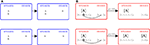Figure 1. Comparison of the basic and Bayesian NG models. (A) Basic two-conventions NG model. In a communication failure (upper figure), the name conveyed, B in the example, is not present in the list of the hearer, who adds it to the list. In a communication success (lower figure), the word B is already present in the hearer's list, and both agents erase A from their lists. (B) Bayesian NG model. In order to convey an example “+” to the hearer in association with name A, the speaker must have already generalized concept C in association with A, represented here by the label [A]. In a communication failure (upper figure), the hearer computes the Bayes probability p, and the result is a p < 1/2; then, the only outcome is that the hearer records the example (reinforcement). In the Bayesian NG, there are two ways in which the communication can be successful. The first way (lower figure) is when p ≥ 1/2: the hearer generalizes C in association with A and attaches the label [A] to the inventory. The second way (not shown) is the agreement process, analogous to that of the basic NG, when both agents had already generalized concept C in association with name A and remove label [B] from their lists, if present. See text for further details.

### 2.2. Toward a Bayesian Naming Game Model

From a cognitive perspective, a “communication failure” of the NG model can be understood as a learning process in which the hearer learns a new word. It is a “one-shot learning process” because it takes place instantaneously (in a single time step) and independently of the agent's history (i.e., of the previous knowledge of the agent). However, modeling an actual learning process should take into account the agents' experience, based on previous observations (the data already acquired) as well as the uncertain/incomplete character naturally accompanying any learning process.

Here, the one-shot learning is replaced by a process that can describe basic but realistic situations, such as the prototypical “linguistic games” . For example, consider a “lecture game,” in which a lecturer (speaker) utters the name A of an object and shows a real example “+” of the object to a student (hearer), repeating this process a few times. Then, the teacher can e.g., (a) show another example and ask the student to name the object, (b) utter the same name and ask the student to show an example of that object, or (c) do both things (uttering the name and showing the object) and asking the student whether the name↔object correspondence is correct. The student will not be able to answer correctly if they have not received some examples enabling them to generalize the concept C corresponding to the object in association with name A. To model these and similar learning processes, we need a criterion enabling the hearer to assess the degree of equivalence between the new example and the examples recorded previously.

The starting point for the replacement of the one-shot learning is Bayes' theorem. According to Bayes' theorem, the posterior probability p(h|X) that the generic hypothesis h is the true hypothesis, after observing new evidence X, reads [39, 40],

Here, the prior probability p(h) gives the probability of occurrence of the hypothesis h before observing the data, and p(X|h) gives the probability of observing X if h is given. Finally, p(X) gives the normalization constraint; in applications, it can be evaluated as $p\left(X\right)=\sum _{{h}^{\prime }}p\left(X|{h}^{\prime }\right)p\left({h}^{\prime }\right)$, where {h′} ∈ H represents the set of hypotheses, within the hypothesis space H.

The next step is to find a way to compute explicitly the posterior probability p(h|X) through a representation of the concepts and their relative examples in a suitable hypothesis space H of the possible extensions of a given concept C, constituted by the mutually exclusive and exhaustive hypotheses h. Following the experimentally verified Bayesian statistical framework of Tenenbaum [10, 31], we adopt the paradigmatic representation of a concept as a geometrical shape. For example, the concept of the “healthy level” of an individual in terms of the levels of cholesterol x and insulin y, defined by the ranges xaxxb and yayyb, where xi and yi (i = a, b) are suitable values, represents a rectangle in the Euclidean x-y plane ℝ2. Examples of healthy levels of specific individuals 1, 2, … correspond to points $\left({x}_{1},{y}_{1}\right),\left({x}_{2},{y}_{2}\right),\cdots \in {ℝ}^{2}$. In the following, we assume that a hypothesis h is represented by an axis-parallel rectangular region in ℝ2. Figure 2 shows four positive examples, denoted by the symbol “+,” associated to four different points of the plane, consistent with (i.e., contained in) three different hypotheses, shown as rectangles.

FIGURE 2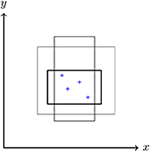Figure 2. Three different hypotheses represented as axis-parallel rectangles in ℝ2, and four positive examples “+” that are all consistent with the three hypotheses. The set of all the rectangles that can be drawn in the plane constitutes the hypothesis space H.

The problem of learning a word is now recast into an equivalent problem, consisting of acquiring the ability to infer whether a new example z recorded, corresponding to a new point “+” in ℝ2, corresponds to the concept C after having seen a small set of positive examples “+” of C. More precisely, let X = {(x1, y1), …, (xn, yn)} be a sequence of n examples of the true concept C, already observed by the hearer, and z = (z1, z2) the new example. The learner does not know the true concept C, i.e., the exact shape of the rectangle associated to C, but can compute the generalization function p(zC|X) by integrating the predictions of all hypotheses h, weighted by their posterior probabilities p(h|X):

Clearly, p(zC|h) = 1 if zh and 0 otherwise. By means of Bayes' theorem (1), one can obtain the right Bayesian probability for the problem at hand. A successful generalization is then defined quantitatively by introducing a threshold p*, representing an acceptance probability: an agent will generalize if the Bayesian probability p (zC|X) ≥ p*. The value p* = 1/2 is assumed, as in Tenenbaum .

We assume that an Erlang prior characterizes the agents' background knowledge. For a rectangle in ℝ2 defined by the tuple (l1, l2, s1, s2), where l1, l2 are the Cartesian coordinates of its lower-left corner and si its sides along dimension i = 1, 2, the Erlang prior density is Tenenbaum [10, 31]

where the parameters σi represent the actual sizes of the concept, i.e., they are the sides of the concept rectangle C along dimension i. The choice of a specific informative prior, such as the Erlang prior, is well motivated by the fact that, in the real world, individuals always have some prior knowledge or expectation. In fact, a Bayesian learning framework with an Erlang prior of the form (3) well describes experimental observations of the learning processes of human beings . The final expression used below for computing the Bayesian probability p that, given the set of previous examples X, the new example z falls in the same category of concept C, reads .

Here, ri (i = 1, 2) is an estimate of the extension of the set of examples along direction i, given by the maximum mutual distance along dimension i between the examples of X; ${\stackrel{~}{d}}_{i}$ measures an effective distance between the new example z and the previously recorded examples, i.e., ${\stackrel{~}{d}}_{i}=0$ if zi falls inside the value range of the examples of X along dimension i, otherwise ${\stackrel{~}{d}}_{i}$ is the distance between z and the nearest example in X along the dimension i. Equation (4) is actually a “quick-and-dirty” approximation that is reasonably good, except for n ≤ 3 and ri ≤ σ/10, estimating the actual generalization function within a 10% error; see Tenenbaum [10, 31] for details. Despite these approximations, Equation (4) will ensure that our computational model, described in the next section, retains the main features of the Bayesian learning framework. It is to be noticed that, for the validity of the Bayesian framework, it is crucial that the examples are drawn randomly from the concept (strong sampling assumption), i.e., they are extracted from a probability density that is uniform in the rectangle corresponding to the true concept . This definition of generalization is now applied below to word-learning.

### 2.3. The Bayesian Word-Learning Model

Based on the Bayesian learning framework discussed above, in this section we introduce a minimal Bayesian individual-based model of word-learning. For the sake of clarity, in analogy with the basic NG model, we study the emergence of consensus in the simple situation, in which two names A and B can be used for referring to the same concept C in pair-wise interactions among N agents.

At variance with the NG model, here, in each basic pair-wise interaction, an agent i, acting as a speaker, conveys an example “+” of concept C, in association with either name A or name B, to another agent j, who acts as hearer (i, j = 1, …, N). In order to be able to communicate concept C by uttering a name, e.g., name A, the speaker i must have already generalized concept C in association with name A. This is signaled by the presence of name A in list ${L}$i. On the other hand, the hearer j always records the example received in the respective inventory, in the example, the inventory [+ + + …]A.

The state of a generic agent i at time t is defined by:

• List ${L}$i, to which a name is added whenever agent i generalizes concept C in association with that name; agent i can use any name in ${L}$i to communicate C;

• Two inventories [+ + + …]A and [+ + + …]B containing the examples “+” of concept C received from the other agents in association with name A and B, respectively.

It is assumed that, initially, each agent knows one word: a fraction nA(0) of the agents know concept C in association with name A, and the remaining fraction nB(0) = 1 − nA(0) in association with name B—no agent knows both words, nAB(0) = 0. We will examine three different initial conditions:

Initially, each agent i, within the fraction nA(0) of agents that know name A is assigned nex, A = 4 examples “+” of concept C in association with name A but no examples in association with the other name B, so that agent i has an A-inventory [+ + + +]A and an empty B-inventory [·]B. The complementary situation holds for the other agents that know only name B, who initially receive nex, B = 4 examples of concept C in association with name B but none in association with A. This choice, somehow arbitrary, is dictated by the condition that (Equation 4) becomes a good approximation for n > 3 .

Examples are points uniformly generated inside the fixed rectangle corresponding to the true concept C, here assumed to be a rectangle with lower-left corner coordinates (0, 0) and sizes σ1 = 3 and σ2 = 1 along the x- and y-axis, respectively. Results are independent of the assumed numerical values; in particular, no appreciable variation in the convergence times tconv is observed as the rectangle area is varied, which is consistent with the strong sampling assumption on which the Bayesian learning framework rests; see Tenenbaum  and section 3.

Furthermore, we introduce an element of asymmetry between the names A and B, related to the word-learning process: different minimum numbers of examples ${n}_{ex,A}^{*}=5$ and ${n}_{ex,B}^{*}=6$ will be used, which are needed by agents to generalize concept C in association with A and B, respectively. This is equivalent to assuming that concept C is slightly easier to learn in association with name A than B. Such an asymmetry plays a relevant role in the model dynamics in differentiating the Bayesian generalization functions pA and pB from each other; see section 4.

The dynamics of the model can be summarized by the following dynamical rules:

1. A pair of agents i and j, acting as speaker and hearer, respectively, are randomly chosen among the agents.

2. The speaker selects randomly (a) a name from the list ${L}$i (or selects the name present if ${L}$i contains a single name), for example, A (analogous steps follow if the word B is selected); (b) an example z among those contained in the corresponding inventory [+ + + …]A;

then the speaker i conveys the example extracted z in association with (e.g., uttering) the name selected A to the hearer j.

3. The hearer adds the new example z (in association with A) to the inventory [+ + + …]A. This reinforcement process of the hearer's knowledge always takes place.

4. Instead, the next step depends on the state of the hearer:

(a) Generalization. If the selected name, A in the example, is not present in the hearer's list ${L}$j, then the hearer j computes the relative Bayesian probability pA = p(zC|XA) that the new example z falls in the same category of concept C, using the examples previously recorded in association with A, i.e., from the set of examples XA ∈ [+ + + …]A. If pA ≥ 1/2, the hearer has managed to generalize concept C and connects the inventory [+ + + …]A to name A; this is done by adding name A to list ${L}$j. Starting from this moment, agent j can communicate concept C to other agents by conveying an example taken from the inventory [+ + + …]A while uttering the name A. If pA < 1/2, the hearer has not managed to generalize the concept and nothing more happens (the reinforcement of the previous point is the only event taking place).

(b) Agreement. The name uttered by the speaker, A in the example, is present in the hearer's list ${L}$j, meaning that agent j has already generalized concept C in association with name A and has connected the corresponding inventory [+ + + …]A to A. In this case, the hearer and the speaker proceed to make an agreement, analogous to that of the NG model, leaving A in their lists ${L}$i and ${L}$j and removing B, if present. No examples contained in any inventory are removed.

5. Time is updated, tt + 1, and the simulation is reiterated from the first point above.

Two examples of the Bayesian word-learning process, a successful and an unsuccessful one, are illustrated in the cartoon in the Figure 1B. Table 1 lists the possible encounter situations, together with the corresponding relevant probabilities.

TABLE 1

Notice that an agent i can enter a pair-wise interaction with a non-empty inventory of examples, e.g., [+ + + …]A, associated to name A, without being able to use name A to convey examples to other agents, i.e., without having the name A in list ${L}$i due to not having generalized concept C in association with A. Those examples can have different origins: (1) in the initial conditions, when nex, A randomly extracted examples associated to A and nex, B to B are assigned to each agent; (2) in previous interactions, in which the examples were conveyed by other agents; (3) in an agreement about convention B, which removed label A from list ${L}$i while leaving all the corresponding examples in the inventory associated to name A. In the latter case, the inventory [+ + + …]A may be “ready” for a generalization process, since it contains a sufficient number of examples, i.e., agent i will probably be able to generalize as soon as another example is conveyed by an agent. This situation is not as peculiar as it may look at first sight. In fact, there is a linguistic analog in the case where a speaker that loses the habit of using a certain word (or a language) A can regain it promptly if exposed to A again.

Notice also that without the agreement dynamics scheme introduced in the model, borrowed from the basic NG model, the population fraction nAB of individuals who know both A and B (nA + nB + nAB = 1) would be growing, until eventually nAB = 1.

## 3. Results

In this section, we numerically study the Bayesian NG model introduced above and discuss its main features. We limit ourselves to studying the model dynamics of a fully-connected network.

In the new learning scheme, which replaces the one-shot learning of the two-conventions NG model, an individual generalizes concept C on a suitable time scale Δt > 1 rather than during a single interaction. However, a few examples are sufficient for an agent to generalize concept C, as in a realistic concept-learning process. This is visible from the Bayesian probabilities pA and pB computed by agents in the role of hearer, according to Equation (4), once at least ${n}_{ex,A}^{*}=5$ and ${n}_{ex,B}^{*}=6$ examples “+”, respectively, have been stored in the inventories associated with the names A and B: Figure 3 shows the histograms of the pAs and pBs computed from the initial time until consensus for a single run with N = 2000 agents and starting with SIC. The low frequencies at small values of pA and pB and the highest frequencies at values close to unity are due to the fact that the Bayesian probabilities reach values pApB ≈ 1 very fast, after a few learning attempts, consistently with the size principle, on which the Bayesian learning paradigm, and in turn Equation (4), are based .

FIGURE 3Figure 3. Histograms of the Bayesian probabilities pA, pB computed by agents during their learning attempts during a single run (for N = 2000 agents, starting with SIC; ${n}_{ex,A}^{*}=5$, ${n}_{ex,B}^{*}=6$).

In order to visualize how the system approaches consensus, it is useful to consider some global observables, such as the fractions nA(t), nB(t), and nAB(t) of agents that have generalized concept C in association with name A only, name B only, or both names A and B, respectively, or the success rate S(t). The dynamics of a population of N = 1, 000 agents (Figures 4A,B) using different initial conditions, SIC, AIC, and AICr, and that of a population of N = 100 agents starting with SIC (Figures 4C,D) are shown in Figure 4.

FIGURE 4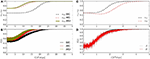Figure 4. Average population fraction associated to the name shared in the final consensus state (A,C) and success rate S(t) (B,D) vs. time. (A,B) System with N = 1, 000 agents starting from different initial conditions, SIC, AIC, and AICr; averages obtained over 600 runs. (C,D) System with N = 100 agents starting from SIC; averages obtained over 1, 000 runs—notice that due to the smaller size N = 100, the system can converge to consensus both with name A (in a fraction of cases pe,A ≈ 0.9) and with name B (pe,B ≈ 0.1).

Figure 4A shows only the population fractions corresponding to the name found at consensus, for the sake of clarity (the remaining population fractions eventually go to zero). For an asymmetrical initial condition (AIC or AICr), it is the initial majority that determines the convention found at consensus (that is, B for AIC and A for AICr). If the system starts from SIC, convention A, for which agents can generalize earlier (${n}_{ex,A}^{*}=5<{n}_{ex,B}^{*}=6$), is always found at consensus—in this case, it is the asymmetry in the thresholds ${n}_{ex,A}^{*}$ and ${n}_{ex,B}^{*}$, characterizing the Bayesian learning process, that determine consensus.

Figure 4B shows the success rate S(t = tk), representing the average over different runs of the instantaneous success rate Sk of the kth interaction at time tk, defined as follows: Sk = 1 in case of agreement between the two agents or when successful learning by the hearer takes places, following a Bayes probability p ≥ 1/2; or Sk = 0 in case of unsuccessful generalization, when p < 1/2 and only reinforcement takes place. The success rate S(t) varies between $S\left(0\right)\approx {\left({n}_{A}\left(0\right)\right)}^{2}+{\left({n}_{B}\left(0\right)\right)}^{2}$, due to the respective fractions of agents that initially know the two conventions A and B, to S ≈ 1 at consensus, following a typical S-shaped curve of learning processes . In the case of SIC, the initial value is S(0) ≈ 0.52 + 0.52 = 0.5, while for AIC or AICr the initial value is S(0) ≈ (0.3)2 + (0.7)2 ≈ 0.58.

We now investigate how the modified Bayesian dynamics affects the convergence times to consensus. The study of the size-dependence of the convergence to consensus shows that there is a critical value N* ≈ 500 in the case of SIC, such that for NN*, there is a non-negligible probability that the final absorbing state is B. Figures 4C,D, representing the results for a system starting with SIC and a smaller size N = 100, show the existence of two possible final absorbing states, and that there are different timescales associated with the convergence to consensus: name A is found at consensus in about 90% of cases and name B in the remaining cases. The branching probability into A or B consensus is further investigated in Figure 5A, where we plot the branching probabilities pe,A, pe,B vs. the system sizes N. The nonlinear behavior (symmetrical sigmoid) signals the presence of finite-size effects, which are particularly clear for relatively small N-values. In fact, when the fluctuations in the system are larger, the system size can play an important role in the dynamics of social systems, as an actual thermodynamic limit is only allowed for simulations of macroscopic physical systems .

FIGURE 5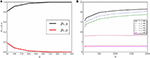Figure 5. (A) Probabilities pe,A and pe,B that the system reaches consensus at A and B respectively, vs. the system size N, obtained by averaging over 1, 000 runs of a system starting with SIC. (B) Average number of examples ${\stackrel{̄}{n}}_{ex,A}$ and ${\stackrel{̄}{n}}_{ex,B}$ recorded by an agent at consensus for a system of N = 50, 100, 500, 1,000, 1,500, or 2,000 agents, starting with SIC, AIC, and AICr. Averages are obtained over 600 runs.

The convergence time tconv follows a simple scaling rule with the system size N, related to the average number of examples ${\stackrel{̄}{n}}_{ex,A},{\stackrel{̄}{n}}_{ex,B}$ relative to A, B respectively, stored in the agents' inventories at consensus. These values depend on the number of learning and reinforcement processes, and hence are related to the system size N. The average number of interactions undergone by the agents until the system reaches the consensus is given by the sum ${\stackrel{̄}{n}}_{int}={\stackrel{̄}{n}}_{ex,A}+{\stackrel{̄}{n}}_{ex,B}$1. One expects that:

which suggests a linear scaling law (tconv ~ N) for convergence time with the system size N for all the possible initial conditions. Linear behavior is indeed confirmed by the numerical simulations with population sizes N = 50, 100, 500, 1,000, 1,500, and 2,000 starting from SIC, AIC, and AICr. The relative numerical results are reported in Table 2. Moreover, in Equation (5) the size-dependence of ${\stackrel{̄}{n}}_{int}$ is ignored as it shows a weak dependence upon N; see Figure 5B.

TABLE 2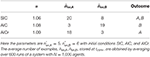Table 2. Scaling laws ${t}_{conv}~{N}^{\alpha }$ with the system size N.

From the above-mentioned scaling law, it is clear that the average number of examples stored by the agents at consensus plays an important role in the semiotic dynamics. In particular, it is found that if the final absorbing state is A (or B), then ${\stackrel{̄}{n}}_{ex,A}>{\stackrel{̄}{n}}_{ex,B}$ (${\stackrel{̄}{n}}_{ex,B}>{\stackrel{̄}{n}}_{ex,A}$). Moreover, the average number of examples, relative to the absorbing state, always increases monotonically with the system size while a size-independent behavior is observed in the opposite case; see Figure 5B.

Finally, we compare the convergence time of the Bayesian word-learning model, tconv, with that of the two-conventions NG model, ${\stackrel{̄}{t}}_{conv}$  by studying the corresponding ratio $R={t}_{conv}/{\stackrel{̄}{t}}_{conv}$ for common initial conditions and population sizes. When starting with SIC, the values of the convergence times obtained from the two models become of the same order by increasing N: R decreases with N, reaching unity for N = 10, 000; see Figure 6. In other words, the time scales of the two models become equivalent for relatively large system sizes, i.e., the learning processes of the two models perform equivalently and the Bayesian approach roughly gives rise to the one-shot learning that characterizes the two-conventions NG model. In the next section, we discuss how the Bayesian model becomes asymptotically equivalent to the minimal NG model. The inset of Figure 6 represents R vs. N for N < 2000, given different starting configurations, with SIC, AIC, and AICr, and different population sizes.

FIGURE 6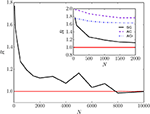Figure 6. The ratio of the convergence times of the Bayesian word-learning model and the two-conventions NG model, $R={t}_{conv}/{\stackrel{̄}{t}}_{conv}$ vs. the system size N for a system starting with SIC. The inset illustrates the dependence of R on different initial conditions. The curves are obtained by averaging over 900 runs.

## 4. Stability Analysis

In this section, we investigate the stability and convergence properties of the mean-field dynamics of the Bayesian NG model, in which statistical fluctuations and correlations are neglected.

### 4.1. Mean-Field Equations

In the Bayesian NG model, as in the basic NG, agents can use two non-excluding options A and B to refer to the same concept C. The main difference between the Bayesian model and the basic NG model is in the learning process: a one-shot learning process in the basic NG and a Bayesian process in the Bayesian NG model. In the latter case, the presence of a name in the word list indicates that the agent has generalized the corresponding concept from a set of positive recorded examples.

The NG model belongs to the wide class of models with two non-excluding options A and B, such as many models of bilingualism , in which transitions between state (A) and state (B) are allowed only through an intermediate (“bilingual”) state (A, B), as schematized in Figure 7. The mean-field equations for the fractions nA(t) and nB(t) can be obtained by considering the gain and loss contributions of the transitions depicted in Figure 7,

Here, $\stackrel{\bullet }{{n}_{a}}\left(t\right)=d{n}_{a}\left(t\right)/dt$ and the quantities pab represent the respective transition rates per individual, corresponding to the arrows in Figure 7 (a, b = A, B, AB). The equation for nAB(t) was omitted, since it is determined by the condition that the total number of agents is constant, nA(t) + nB(t) + nAB(t) = 1.

FIGURE 7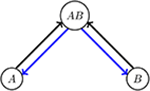Figure 7. Model scheme with two non-excluding options. Arrows indicate allowed transitions between the “bilingual” state (A,B) and the “monolingual” states A and B. Direct AB transitions are not allowed.

The details of the possible pair-wise interactions in the Bayesian naming game are listed in Table 1. By adding the various contributions, one obtains the equation for the average population fractions,

which can be rewritten in the form (6) with transition rates per individual given by:

Equations (8) provide the transition rates of learning processes, while Equations (9) give the transition rates of agreement processes.

In the rest of the paper, we set xnA, ynB, and z = nAB ≡ 1 − xy, so that the autonomous system (7) becomes:

in which we have defined the velocity field v = (fx(x, y), fy(x, y)) in the phase plane. The Bayesian probabilities pA and pB appear in these equations as time-dependent parameters of the model, but they are actually highly non-linear functions of the variables. In fact, they can be thought as averages of the microscopic Bayesian probability in Equation (4) over the possible dynamical realizations. For this reason, they have also a complex non-local time-dependence on the previous history of the interactions between agents. For the moment, we assume pA(t) = pB(t) = p(t), returning later to the general case.

From the conditions defining the critical points, fx(x, y) = fy(x, y) = 0, one obtains (xy)z = 0. Setting z = 0, one obtains two solutions that correspond to consensus in A or B, given by (x1, y1, z1) = (1, 0, 0) and (x2, y2, z2) = (0, 1, 0). Instead, setting (xy) = 0 leads to the equation:

which has the solutions,

One can check that for every value of p ∈ (0, 1], the corresponding solutions (x±, x±, 1 − 2x±) are not suitable solutions, because z± = 1 − 2x± < 0.

This analysis is valid for p > 0. In fact, p = p(t) is a function of time and, for a finite interval of time after the initial time, one has that p = 0, which defines a different dynamical system: the transition from p = 0 to p > 0 is accompanied by a bifurcation, as becomes clear by analyzing the equilibrium points. In the initial conditions used, z(0) = 0, which implies z(t) = 0, x(t) = x(0), and y(t) = y(0) at any later time t as long as p(t) = 0, since (t) = (t) = ż(t) = 0 (see Equation 7); in fact, the whole line x + y = 1 (for 0 < x, y < 1) represents a continuous set of equilibrium points. The reason why, in this model, p(0) = 0 at t = 0 and also during a subsequent finite interval of time is twofold. First, agents do not have any examples associated to the name not known, and they have to receive at least ${n}_{ex,A}^{*}$ or ${n}_{ex,B}^{*}$ examples before being able to compute the corresponding Bayesian probability pA(t) or pB(t)—thus, it is to be expected that p(t) = 0 meanwhile. Furthermore, even when agents can compute the Bayesian probabilities, the effective probability to generalize is actually zero, due to the threshold p* = 0.5 for a generalization to take place. The existence of the (temporary) equilibrium points on the line x + y = 1 ends as soon as the parameter p(t) > p* and, according to Equation (7), a bifurcation takes place: the two A- and B-consensus states become the only stable equilibrium points, and the representative point in the x-y-plane is deemed to leave the initial conditions on the z = 1 − xy = 0 line due to the stochastic nature of the dynamics, which is not invariant under time reversal .

To determine the nature of the critical points (x1, y1) = (1, 0) and (x2, y2) = (0, 1), one needs to evaluate at the equilibrium points the 2 × 2 Jacobian matrix A(x, y) = {∂ifj}, where i, j = x, y. Equations (10, 11) give:

whose eigenvalues at a given time t are λ1 = [p(t) − 3]/2 and λ2 = − p(t). As they are both negative and distinct for 0 < p ≤ 1, λ1 < λ2 < 0, the critical points (0, 1) and (1, 0) are asymptotically stable . It can be easily checked that these conclusions are unchanged if the generalization probabilities are different, pA(t)≠pB(t). For instance, one would have:

associated to eigenvalues with different numerical values but the same sign, not changing the nature of the critical point. Thus, the asymptotically stable nodes (x1, y1) = (1, 0) and (x2, y2) = (0, 1) are the only absorbing states of the Bayesian naming game.

### 4.2. A Geometric Analysis of Consensus

We consider a system starting from SIC, defined by the point (x0, y0) = (0.5, 0.5) located on the line x + y = 1 of the phase plane, representing a system that initially has 50% of agents with list (A), 50% with list (B), and no agent with list (A, B).

From Equation (7) and the fact that, initially, pA(0) = pB(0) = 0 (and z(0) = 0), one can see that the corresponding velocity is v(0) = (fx(x(0), y(0)), fy(x(0), y(0))) = (0, 0), meaning that the initial SIC state (x(0), y(0)) = (0.5, 0.5) is a temporary equilibrium point. As soon as pA(t), pB(t) > 0, at a time t = t* > 0, the velocity becomes different from zero, and the representative point (x(t), y(t)) moves away in the phase plane with velocity v(t) = (fx(x(t), y(t)), fy(x(t), y(t))). The system is observed to eventually reach either A-consensus at (x1, y1) = (1, 0) or B-consensus at (x2, y2) = (0, 1). These two types of evolution are illustrated in Figure 8 through the population fractions nA(t) and nB(t) vs. time t taken from two single runs of a population with size N = 100 agents.

FIGURE 8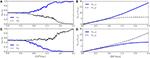Figure 8. Results from two single simulations of a system with N = 100 agents, starting from SIC at (x0, y0) = (0.5, 0.5) and reaching two different consensus states about name A or B. (A,C) Show the population fractions x(t) = nA(t) and y(t) = nB(t). (B,D) Show the corresponding average number of examples recorded by an agent, ${\stackrel{̄}{n}}_{ex,A}\left(t\right)$ and ${\stackrel{̄}{n}}_{ex,B}\left(t\right)$.

In order to determine the conditions for this to happen, we evaluate the scalar product between the velocity v(t*) at time t* and the versor $\text{u}=\left(1/\sqrt{2},-1/\sqrt{2}\right)$, parallel to the line x + y = 1 and directed toward the A-consensus state (1, 0); see Figure 9. If the velocity vector v has a positive component along u, the representative point will move from the initial state (0.5, 0.5) toward the A-fixed point (1, 0); instead, in the case of a velocity vector v′ with a negative component along u, the representative point will move toward the B-fixed point (1, 0); see Figure 9. From the simulations, we know that during the initial transient, the population dynamics is characterized by a nA(t) ≈ nB(t), until the critical time t = t* is reached. This allows one to set xy in this interval of time—this phenomenon is a sort of stiffness of the system before starting to explore the phase plane. The scalar product u · v, where the velocity vector's components are given explicitly in Equation (7), is positive at t = t* when:

which gives the condition:

Equation (17) clearly shows that the values of the Bayesian probabilities pA, pB become different at the critical time t*, thus allowing the solutions to split into different orbits, going toward the state of consensus in A and B. The same reasoning, applied to the case of an orbit bending toward the consensus state in B at (0, 1), would give ${p}_{A}\left({t}^{*}\right)<{p}_{B}\left({t}^{*}\right)$. The difference between the probabilities pA and pB can be traced back to the way the generalization function p(t) is used by agents to compute either pA(t) or pB(t). In fact, the value of p(t) depends on the examples recorded, which constitute the inputs of the function. We argue that this behavior is due to the fluctuations of the numbers of examples ${\stackrel{̄}{n}}_{ex,A}\left(t\right)$ and ${\stackrel{̄}{n}}_{ex,B}\left(t\right)$, recorded by the agents until time t, together with the initial asymmetry of the thresholds for generalizing, ${n}_{ex,A}^{*}\ne {n}_{ex,B}^{*}$. In fact, the stochastic nature of the pairwise-interactions leads to different examples (that can be better or worse for the aim of generalizing) and to different inventory sizes, i.e., the numbers of examples stored by agents at time t; clearly all this strongly affects the path to consensus. Furthermore, asymmetrical thresholds (${n}_{ex,A}^{*}=5<{n}_{ex,B}^{*}=6$ were used) produce a bias favoring consensus in A and play a crucial role in the subsequent Bayesian semiotic dynamics, letting concept C be learned more often in association with A than B and contributing to making consensus in A more frequent: swapping the threshold values (setting ${n}_{ex,A}^{*}=6>{n}_{ex,B}^{*}=5$), the approach to consensus occurs with the outcomes A, B swapped.

FIGURE 9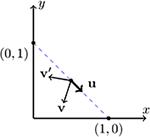Figure 9. The vectors v and v′ represent two possible velocities emerging from the point (x0, y0) = (0.5, 0.5) at t = t*. The unit vector u is directed along the line x + y = 1 joining the asymptotically stable nodes (x1, y1) = (1, 0) and (x2, y2) = (1, 0).

For NN* ≈ 500, the chances that the system converges to (B) become negligible. This can be seen in of Figures 8B,D, showing ${\stackrel{̄}{n}}_{ex,A}\left(t\right)$ and ${\stackrel{̄}{n}}_{ex,B}\left(t\right)$ vs. time (averaged over the agents of the system) for a single run of a system with a population of N = 100 agents, starting with SIC. Panels (B) and (D) compare the results obtained from selected runs ending at consensus A and B, respectively. It is evident that, after an initial transient, in which ${\stackrel{̄}{n}}_{ex,A}\left(t\right)\approx {\stackrel{̄}{n}}_{ex,B}\left(t\right)$, they start to differ more and more significantly from each other at times t > t*. In turn, starting from this point, also pA and pB begin to differ significantly from each other, thus affecting the rate of depletion of the populations during the subsequent dynamics. For instance, if pA > pB, then pBAB > pAAB (see Equations (8)), which means that the depletion of nB occurs faster then that of nA. In turn, this favors the decay of the mixed states (A, B) into the state (A) (see Equations (9)), being nA > nB.

The asymmetry discussed above, about the rate of convergence toward the final consensus states, also affects the values of convergence times ${t}_{conv}^{A}$ and ${t}_{conv}^{B}$ needed for a system to reach consensus at A and B, respectively: we find ${t}_{conv}^{B}>{t}_{conv}^{A}$ in all the numerical simulations. The difference in the convergence times is already appreciable, despite the noise, in the output of a single run, such as the population fractions shown in Figures 8A,C. Mean fractions nA(t), nB(t), and nAB(t) vs. time, obtained by averaging over many runs, result in less noisy outputs and provide a more clear picture of the difference, which is visible in Figure 10, obtained using 600 runs starting with SIC and for N = 100 agents (Figures 10A,C) and N = 200 agents (Figures 10B,D). In addition, one can notice that the convergence times strongly depend on the system size: increasing the number of agents N slows down the relaxation, and both the times ${t}_{conv}^{A}$ and ${t}_{conv}^{B}$ increase, as is evident by comparing the (Figures 10A,C) (N = 100 agents) with the (Figures 10B,D) (N = 200 agents).

FIGURE 10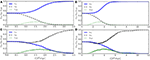Figure 10. Population fractions nA(t), nB(t), and nAB(t) vs. time, starting from SIC; results are obtained by averaging over 600 runs. Left column (A,C): a system with N = 100 agents can reach consensus with name A (A, about 91% of runs) or name B (C, about 9% of runs). Right column (B,D): system with N = 200 agents, reaching consensus with name A in about 96% of runs (B) and with name B in about in the remaining 4% of runs (D).

The possibility that a system starting with the same initial conditions and with the same parameters can reach both consensus states is a consequence of the stochastic nature of the pairwise-interactions, together with the asymmetry in the threshold values ${n}_{ex,A}^{*}=5$ and ${n}_{ex,B}^{*}=6$. It stops occurring for NN*, when both ${\stackrel{̄}{n}}_{ex,A}$ and ${\stackrel{̄}{n}}_{ex,B}$ reach some threshold values close to those observed at tconv, which is clearly a value sufficient for the agents to generalize concept C. In fact, the scaling law of tconv with N shows that the sum of ${\stackrel{̄}{n}}_{ex,A}$ with ${\stackrel{̄}{n}}_{ex,B}$ becomes nearly constant for NN*, implying that the dynamics is uniquely determined, that is, the consensus always occurs at A from SIC, once the agents have stored a threshold number of ${\stackrel{̄}{n}}_{ex,A}$, ${\stackrel{̄}{n}}_{ex,B}$. It is found that these threshold values correspond to ${\stackrel{̄}{n}}_{ex,A}=21$, ${\stackrel{̄}{n}}_{ex,B}=12$. Note that, to the latter values ${\stackrel{̄}{n}}_{ex,A}$, ${\stackrel{̄}{n}}_{ex,B}$, we also add the four initial given examples stored in the agents' inventories at the beginning. This is because the generalization function p(t) outputs will effectively depend on them all. Therefore, at these threshold values, it would be very unlikely that pB > pA, and so it would be the same for the consensus at B.

Now, we consider some variables that characterize the Bayesian process underlying pair-wise interactions and how they vary with time, in particular the Bayesian probabilities pA(t) and pB(t), computed by agents, and the corresponding number of learning attempts noA(t) and noB(t) made by agents at time t to learn concept C in association with word A or B, respectively, i.e., the number of times that the agents compute pA or pB. Only the case of a system starting with SIC is considered, but the other cases present similar behaviors. We consider a single run of a system with N = 5, 000 agents and study the average values ${\stackrel{̄}{p}}_{A}\left(t\right),{\stackrel{̄}{p}}_{B}\left(t\right)$ obtained by averaging pA(t) and pB(t) over the agents of the system. Furthermore, employing a coarse-grained view, an additional average (of both the probabilities ${\stackrel{̄}{p}}_{A}\left(t\right),{\stackrel{̄}{p}}_{B}\left(t\right)$ and the numbers of attempts noA, noB) over a suitable time-interval (a temporal bin Δt = 16 × 103) reduces random fluctuations. Figure 11 shows the time evolution of the average probabilities ${\stackrel{̄}{p}}_{A}\left(t\right)$ and ${\stackrel{̄}{p}}_{B}\left(t\right)$ in the time-range where data allow good statistics. The probabilities grow monotonically and eventually reach the value one. While this points at an equivalence between the mean-field regime of the Bayesian naming game and that of the two-conventions NG model, in which agents learn at the first attempt (one-shot learning), such an equivalence is suggested but not fully reproduced by the coarse-grained analysis. The time evolution of the number of learning attempts noA(t) and noB(t) shows that they are negligible both at the beginning and at the end of the dynamics—see inset in Figure 11. This is due to the fact that at the beginning it is most likely that either interactions between agents with the same conventions take place (starting with SIC, each agent has a probability of 50% to interact with an agent having the same convention) or that interactions between agents with different conventions but with still too small inventories to be able to generalize concept C take place, leading to reinforcement processes only. When approaching consensus, agents with one of the conventions constitute the large majority of the population, and thus they are again most likely to interact through reinforcements only. Thus, the largest numbers of attempts to learn concept C in association with A and B are expected to occur at the intermediate stage of the dynamics. In fact, noA(t) and noB(t) are observed to reach a maximum at ttconv/2 for any given system size N, as is visible in the inset of Figure 11. Notice that also the fraction of agents nAB who know both conventions and can communicate using both name A and name B, possibly allowing other agents to generalize in association with name A or B, reaches its maximum roughly at the same time.

FIGURE 11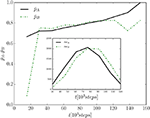Figure 11. Average values ${\stackrel{̄}{p}}_{A}\left(t\right)$ and ${\stackrel{̄}{p}}_{B}\left(t\right)$ computed using a (temporal) bin Δt = 16 × 103 vs. time from for a single run of a system reaching consensus at A. The convergence time is ${t}_{conv}\approx 160×1{0}^{3}$, and the population size is N = 5, 000. The inset shows the average number of learning attempts noA, noB vs. time for the same single run.

## 5. Conclusion

We constructed a new agent-based model that describes the appearance of linguistic consensus through a word-learning process, representing an original example of an opinion dynamics or culture competition model translated at a cognitive level, something that is not apparent. The model represents a Bayesian extension of the semiotic dynamics of the NG model, with an underlying cognitive process that mimics the human learning processes; it can describe in a natural way the uncertainty accompanying the first phase of a learning process, the gradual reduction of the uncertainty as more and more examples are provided, and the ability to learn from a few examples.

The work presented is exploratory in nature, concerning the minimal problem of a concept, C that can be associated to two different possible names A and B. The resulting semiotic dynamics of the synonyms is different from the basic NG, in that it depends on parameters that are strictly cognitive in nature, such as a minimum level of experience (quantified by the number of examples ${n}_{ex}^{*}$ necessary for generalizing) and the threshold for generalizing a concept (represented by a critical value of the Bayesian acceptance probability p*). The interplay between the asymmetry of the conventions A and B, the system size, and the stochastic character of the time evolution have dramatic consequences on the consensus dynamics, leading to a critical time t* > 0 before the system begins to move in the phase-plane, to converge eventually toward a consensus state; a critical system size N*, below which there is an appreciable probability that the system can end up in any of the two possible consensus states and, in general, a dependence of the convergence times on N; an asymmetry in the convergence times and the corresponding branching probabilities that the system converges toward one of the two possible conventions; different scaling of the convergence times vs. N with respect to those observed in the basic NG model, due to the dependence on the learning experience of the agents.

The cognitive dimension of the novel model offers the possibility to study the effects that are out of the reach of other opinion dynamics or cultural exchange models, such as the basic NG model. The corresponding dynamical equations, Equations (10, 11), provide a general mean-field description of a group of individuals communicating with each other while undergoing cognitive processes. The cognitive dynamics are fully contained in the functions pA(t) and pB(t). Similar models but with different or more general underlying cognitive dynamics are expected to leave the form of Equations (10, 11) unchanged, only changing the functional forms of pA(t) and pB(t). In this sense, the model introduced in this work represents a step toward a generalized Bayesian approach to the problem of how social interactions can lead to cultural conventions.

Future work can address specific problems of current interest from the point of view of cognitive processes or features relevant from the general standpoint of complexity theory. In the first case, it is possible to study the semiotic dynamics of homonyms and synonyms, e.g., the problem of a name A1, associated to a concept C1, that at some points splits into two related but distinct concepts C1 and C2, analyzing the cognitive conditions for the corresponding splitting of name A1 into two names A1 and A2, as the two concepts eventually become distinguishable to the agents—this type of problem cannot be tackled within models of cultural competition. In the second case, one can mention the classical problem of the interplay between a central information source (bias) and the local influences of individuals—this time, in a cognitive framework—and the role of heterogeneity. In fact, heterogeneity concerns most of the known complex systems at various levels, from the diversity in the dynamical parameters of, e.g., the different competing names and concepts to that of the agents. The heterogeneity of individuals is known to lead to counter-intuitive effects, such as resonant behaviors [46, 47]. Furthermore, the complex, heterogeneous nature of a local underlying social network can drastically change the co-evolution of the conventions in competition with each other and therefore the relaxation process .

## Data Availability Statement

The raw data supporting the conclusions of this article will be made available by the authors, without undue reservation, to any qualified researcher.

## Author Contributions

GM wrote the codes and performed the numerical simulations. GM, MP, and EH contributed to the design of the numerical experiments, the analysis of the results, and the writing of the manuscript.

## Funding

The authors acknowledge support from the Estonian Ministry of Education and Research through Institutional Research Funding IUT (IUT39-1), the Estonian Research Council through Grant PUT (PUT1356), and the ERDF (European Development Research Fund) CoE (Center of Excellence) program through Grant TK133.

## Conflict of Interest

The authors declare that the research was conducted in the absence of any commercial or financial relationships that could be construed as a potential conflict of interest.

## Acknowledgments

We thank Andrea Baronchelli for providing useful remarks about the naming game model and the manuscript.

## Footnotes

1. ^The nex, A = nex, B = 4 examples given initially to each agent are not accounted for by ${\stackrel{̄}{n}}_{ex,A}$ and ${\stackrel{̄}{n}}_{ex,B}$.

## References

1. Castellano C, Fortunato S, Loreto V. Statistical physics of social dynamics. Rev Mod Phys. (2009) 81:591. doi: 10.1103/RevModPhys.81.591

2. Baronchelli A. The emergence of consensus: a primer. R Soc Open Sci. (2018) 5:172189. doi: 10.1098/rsos.172189

3. Xia C, Ding S, Wang C, Wang J, Chen Z. Risk analysis and enhancement of cooperation yielded by the individual reputation in the spatial public goods game. IEEE Syst J. (2017) 11:1516–25. doi: 10.1109/JSYST.2016.2539364

4. Xia C, Li X, Wang Z, Perc M. Doubly effects of information sharing on interdependent network reciprocity. N J Phys. (2018) 20:075005. doi: 10.1088/1367-2630/aad140

5. Zhang Y, Wang J, Ding C, Xia C. Impact of individual difference and investment heterogeneity on the collective cooperation in the spatial public goods game. Knowledge Based Syst. (2017) 136:150–8. doi: 10.1016/j.knosys.2017.09.011

6. Mufwene S. The Ecology of Language Evolution. Cambridge: Cambridge University Press (2001).

7. Lass R. Historical Linguistics and Language Change. Cambridge: Cambridge University Press (1997).

8. Berruto C. Prima lezione di sociolinguistica. Roma: Laterza (2004).

9. Edelman S, Waterfall H. Behavioral and computational aspects of language and its acquisition. Phys Life Rev. (2007) 4:253–77. doi: 10.1016/j.plrev.2007.10.001

10. Tenenbaum JB. A Bayesian framework for concept learning. Ph.D. thesis, Boston, MA: MIT (1999).

11. Wichmann S. The emerging field of language dynamics. Lang Linguist Compass. (2008) 3:442. doi: 10.1111/j.1749-818X.2008.00062.x

12. Wichmann S. Teaching & learning guide for: The emerging field of language dynamics. Lang Linguist Compass. (2008) 2:1294–7. doi: 10.1111/j.1749-818X.2008.00109.x

13. Tenenbaum JB, Xu F. Word learning as Bayesian inference. In: Proceedings of the 22nd Annual Conference of the Cognitive Science Society Mahwah, NJ (2000).

14. Xu F, Tenenbaum JB. Word learning as Bayesian inference. Psychol Rev. (2007) 114:245–72. doi: 10.1037/0033-295X.114.2.245

15. Hurford J. Biological evolution of the saussurean sign as a component of the language-acquisition device. Lingua. (1989) 77:187–222.

16. Nowak MA, Plotkin JB, Krakauer DC. The evolutionary language game. J Theoret Biol. (1999) 200:147–62. doi: 10.1006/jtbi.1999.0981

17. Nowak M. Evolutionary biology of language. Philos Trans R Soc London B Biol Sci. (2000) 355:1615–22. doi: 10.1098/rstb.2000.0723

18. Trapa PE, Nowak MA. Nash equilibria for an evolutionary language game. J Math Biol. (2000) 41:172–88. doi: 10.1007/s002850070004

19. Chen G, Lou Y. Naming Game. Switzerland: Springer International Publishing (2019).

20. Lenaerts T, Jansen B, Tuyls K, De Vylder B. The evolutionary language game: an orthogonal approach. J Theor Biol. (2005) 235:566–82. doi: 10.1016/j.jtbi.2005.02.009

21. Lipowska D, Lipowski A. Emergence of linguistic conventions in multi-agent reinforcement learning. PLoS ONE. (2018). 13:e0208095. doi: 10.1371/journal.pone.0208095

22. Xie J, Emenheiser J, Kirby M, Sreenivasan S, Szymanski BK, Korniss G. Evolution of opinions on social networks in the presence of competing committed groups. PLoS ONE. (2012) 7:e33215. doi: 10.1371/journal.pone.0033215

23. Fan ZY, Lai YC, Tang WKS. Knowledge consensus in complex networks: the role of learning. tt arXiv:1809.00297 (2018).

24. Eguluz VM, Masuda N, Fernández-Gracia J. Bayesian decision making in human collectives with binary choices. PLoS ONE. (2015) 10:e0121332. doi: 10.1371/journal.pone.0121332

25. Pérez T, Zamora J, Eguíluz VM. Collective intelligence: aggregation of information from neighbors in a guessing game. PLoS ONE. (2016) 11:e0153586. doi: 10.1371/journal.pone.0153586

26. Baronchelli A. A gentle introduction to the minimal naming game. Belg J Linguist. (2016) 30:171–92. doi: 10.1075/bjl.30.08bar

27. Tenenbaum JB, Griffiths TL. Generalization, similarity, and Bayesian inference. Behav Brain Sci. (2001) 24:629–40. doi: 10.1017/S0140525X01000061

28. Griffiths TL, Tenenbaum JB. Optimal predictions in everyday cognition. Psychol Sci. (2006) 17:767–73. doi: 10.1111/j.1467-9280.2006.01780.x

29. Perfors A, Tenenbaum JB, Griffiths TL, Xu F. A tutorial introduction to Bayesian models of cognitive development. Cognition. (2011) 120:302–21. doi: 10.1016/j.cognition.2010.11.015

30. Lake BM, Salakhutdinov R, Tenenbaum JB. Human-level concept learning through probabilistic program induction. Science. (2015) 350:1332–8. doi: 10.1126/science.aab3050

31. Tenenbaum JB. Bayesian modeling of human concept learning. In: Proceedings of the 1998 Conference on Advances in Neural Information Processing Systems II. Cambridge, MA: MIT Press (1999). p. 59–65

32. Tenenbaum JB, Kemp C, Griffiths TL, Goodman ND. How to grow a mind: statistics, structure, and abstraction. Science. (2011) 331:1279–85. doi: 10.1126/science.1192788

33. Barber D. Bayesian Reasoning and Machine Learning. Cambridge: Cambridge University Press (2012).

34. Murphy K. Machine Learning: a Probabilistic Perspective. Cambridge, MA: MIT Press (2012).

35. Evgeniou T, Pontil M, Poggio T. Statistical learning theory: a primer. Int J Comput Vision. (2000) 38:9–13. doi: 10.1023/A:1008110632619.

36. Castelló X, Baronchelli A, Loreto V. Consensus and ordering in language dynamics. Eur Phys J B. (2009) 71:557–64. doi: 10.1140/epjb/e2009-00284-2

37. Baronchelli A, Dall'Asta L, Barrat A, Loreto V. Nonequilibrium phase transition in negotiation dynamics. Phys Rev E. (2007) 76:051102. doi: 10.1103/PhysRevE.76.051102

38. Wittgenstein L. Philosophical Investigations. New York, NY: Macmillan (1953).

39. Harney HL. Bayesian Inference. Parameter Estimation and Decisions. Berlin: Springer (2016).

40. Jeffreys H. Theory of Probability. Oxford: Clarendon Press (1939).

41. Baronchelli A, Felici M, Loreto V, Caglioti E, Steels L. Sharp transition towards shared vocabularies in multi-agent systems. J Statist Mech Theory Exp. (2006) P06014. doi: 10.1088/1742-5468/2006/06/P06014

42. Toral R, Tessone C. Finite size effects in the dynamics of opinion formation. Comm Comp Phys. (2007) 2:177.

43. Patriarca M, Castelló X, Uriarte J, Eguíluz V, San Miguel M. Modeling two-language competition dynamics. Adv Comp Syst. (2012) 15:1250048. doi: 10.1142/S0219525912500488

44. Hinrichsen H. Non-equilibrium phase transitions. Phys A Statist Mechan Appl. (2006) 369:1–28. doi: 10.1016/j.physa.2006.04.007

45. Strogatz SH. Nonlinear Dynamics and Chaos: With Applications to Physics, Biology, Chemistry and Engineering. Northwestern, NW: CRC Press (2015).

46. Tessone C, Toral R. Diversity-induced resonance in a model for opinion formation. Eur Phys J B. (2009) 71:549. doi: 10.1140/epjb/e2009-00343-8

47. Vaz Martins T, Toral R, Santos M. Divide and conquer: resonance induced by competitive interactions. Eur Phys J B. (2009) 67:329–36. doi: 10.1140/epjb/e2008-00437-9

48. Toivonen R, Castelló X, Eguíluz V, Saramäki J, Kaski K, San Miguel M. Broad lifetime distributions for ordering dynamics in complex networks. Phys Rev E. (2009) 79:016109. doi: 10.1103/PhysRevE.79.016109

Keywords: complex systems, language dynamics, bayesian statistics, cognitive models, consensus dynamics, semiotic dynamics, naming game, individual-based models

Citation: Marchetti G, Patriarca M and Heinsalu E (2020) A Bayesian Approach to the Naming Game Model. Front. Phys. 8:10. doi: 10.3389/fphy.2020.00010

Received: 14 June 2019; Accepted: 09 January 2020;
Published: 13 February 2020.

Edited by:

Raul Vicente, Max-Planck-Institut für Hirnforschung, Germany

Reviewed by:

Chengyi Xia, Tianjin University of Technology, China